# Forbidden

Additionally, a 403 Forbidden error was encountered while trying to use an ErrorDocument to handle the request.

# Soci Benemeriti

[singlepic id=75 w=320 h=240 float=]  [singlepic id=76 w=320 h=240 float=]  [singlepic id=77 w=320 h=240 float=]  [singlepic id=78 w=320 h=240 float=]  [singlepic id=79 w=320 h=240 float=]  [singlepic id=80 w=320 h=240 float=]  [singlepic id=81 w=320 h=240 float=]  [singlepic id=82 w=320 h=240 float=]  [singlepic id=83 w=320 h=240 float=]  [singlepic id=84 w=320 h=240 float=]  [singlepic id=85 w=320 h=240 float=]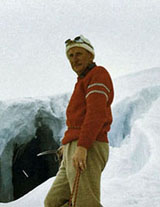LUIGI CORTI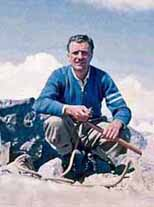DARVINI DELL'ORO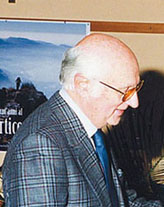SERGIO NECCHI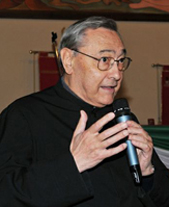DON MASSIMO FRIGERIO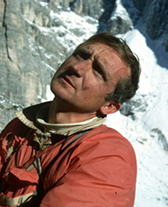GIORGIO REDAELLI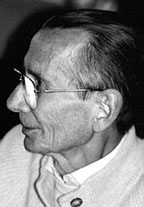PIERO PIACCO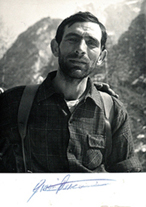GIOVANNI RUSCONIANTONIO RUSCONI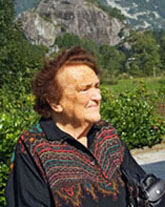VERA CENINI LUSARDI